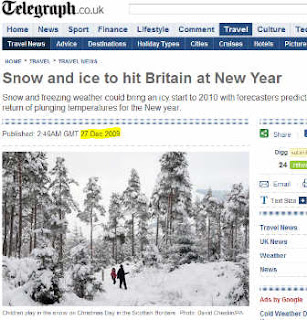## Saturday, 23 January 2010

### The 28 Year Snow and The 100 Year FloodEurope just went through one of its worst winters in years, and according to the press, the largest snowfall in England for 28 years (and apparently similar effects in the US.. that darn El Nino). The last this big in Europe was 1982. That doesn't actually make it a 28 year Snow, in the sense of a 100 year flood. Most people don't realize that the probability of having a "100 year flood" in any 100 years is only about 63% (go on, say "Huh?").. Officially, a 100 year flood is a flood that has a 1/100 chance of happening in any given year (I think, technically, they say the probability of a flood that great or greater). You can use a little easy probability to see why it would only have a 63% probability of occurring in the next 100 years (even if it hasn't occurred in 200 years, or if it happened yesterday.. the assumption is that the great floods are essentially independent events). So the probability of having (at least one) "100 year flood" in the next 100 years is just 1- (the probability that we don't have one)..which is easier to compute. The probability of NOT having a 100 year flood this year is .99 or 1- the probability that we do.. The probability we don't have one in the next 100 years is just (.99)100 which my trusty calculator tells me is about .366. So it there is a .366 chance of NO great flood, then there is a .634 chance of having at least one.
"You mean there might be MORE than one?" I hear you ask. Well yes, now we just apply the binomial distribution to find, for example, the probability of great floods in two of the next 100 years (and not in 98 others) and accounting for all the possible orders, we get $\dbinom{100}{2}(.01)^2(.99)^9^8$ or about 18.5%; and if you extend that to exactly three great floods, the probability comes out to about 6%... so there is something like a 36% chance of NOT having a great flood, and about a 40% chance of just one. (It takes about 230 years to be 90% sure of having a 100 year flood; and almost 300 years to be 95% sure).

Now if we said, Wait, a "100 year flood" should have a probability of almost one of happening (would you settle for the proverbial 95% standard from statistics?) then we could work backwards and compute the probability of such a flood this year (or any random year). Since the probability of not having one in 100 years is 1-.95, all we have to do is solve the problem (1-p)100 = .05 and we get the annual probability of such a flood. Surprisingly, that puts the probability up to about 3% (.0295..)

So if we assume that this winter was the traditional "28 year snow" by the normal definition (ie, one that has a 1/28 chance of occurring) then the probability that it happens again next winter is 3.57% ( a little greater than our "certain 100 year flood". So how likely is THAT to happen in the next 28 years...... (you are soooo gonna love this) .... about 63%, to be slightly more precise, about 63.87%...

Hmmm (wheels turning in your mind)... that's almost the same answer..... "COINCIDENCE? " I ask my students; and they know the proper reply..." I THINK NOT!". So what is happening here. If the probability of an n-year flood is 1/n, then the probability of one occurring in the next n years is given by Prob(flood)=1-$[\frac{n-1}{n}]^n$.. and if we plot this we see that it very quickly becomes asymptotic to about .63... or 1-(1/e); which is perfectly understandable since the limit as n goes to infinity of $[\frac{n-1}{n}]^n$ is e-1; or about .36.Which means, for anything more than a ten year event or so, the probability that it happens in the next N years is always about the same...about 63%... Many questions, one answer.... ahhhh ... gotta love it.